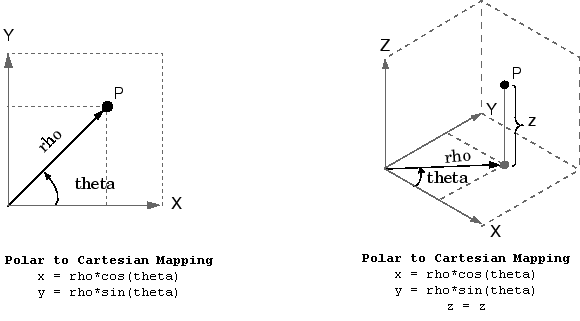# pol2cart

Transform polar or cylindrical coordinates to Cartesian

## Syntax

``````[x,y] = pol2cart(theta,rho)``````
``````[x,y,z] = pol2cart(theta,rho,z)``````

## Description

example

``````[x,y] = pol2cart(theta,rho)``` transforms corresponding elements of the polar coordinate arrays `theta` and `rho` to two-dimensional Cartesian, or xy, coordinates.```

example

``````[x,y,z] = pol2cart(theta,rho,z)``` transforms corresponding elements of the cylindrical coordinate arrays `theta`, `rho`, and `z` to three-dimensional Cartesian, or xyz, coordinates.```

## Examples

collapse all

Convert the polar coordinates defined by corresponding entries in the matrices `theta` and `rho` to two-dimensional Cartesian coordinates `x` and `y`.

`theta = [0 pi/4 pi/2 pi]`
```theta = 1×4 0 0.7854 1.5708 3.1416 ```
`rho = [5 5 10 10]`
```rho = 1×4 5 5 10 10 ```
`[x,y] = pol2cart(theta,rho)`
```x = 1×4 5.0000 3.5355 0.0000 -10.0000 ```
```y = 1×4 0 3.5355 10.0000 0.0000 ```

Convert the cylindrical coordinates defined by corresponding entries in the matrices `theta`, `rho`, and `z` to three-dimensional Cartesian coordinates `x`, `y`, and `z`.

`theta = [0 pi/4 pi/2 pi]'`
```theta = 4×1 0 0.7854 1.5708 3.1416 ```
`rho = [1 3 4 5]'`
```rho = 4×1 1 3 4 5 ```
`z = [7 8 9 10]'`
```z = 4×1 7 8 9 10 ```
`[x,y,z] = pol2cart(theta,rho,z)`
```x = 4×1 1.0000 2.1213 0.0000 -5.0000 ```
```y = 4×1 0 2.1213 4.0000 0.0000 ```
```z = 4×1 7 8 9 10 ```

## Input Arguments

collapse all

Angular coordinate, specified as a scalar, vector, matrix, or multidimensional array. `theta`, `rho`, and `z` must be the same size, or have sizes that are compatible (for example, `theta` is an `M`-by-`N` matrix, `rho` is a scalar, and `z` is a scalar or `1`-by-`N` row vector). For more information, see Compatible Array Sizes for Basic Operations.

`theta` is the counterclockwise angle in the x-y plane measured in radians from the positive x-axis.

Data Types: `single` | `double`
Complex Number Support: Yes

Radial coordinate, specified as a scalar, vector, matrix, or multidimensional array. `theta`, `rho`, and `z` must be the same size, or have sizes that are compatible.

`rho` is the distance from the origin to a point in the x-y plane.

Data Types: `single` | `double`
Complex Number Support: Yes

Elevation coordinate, specified as a scalar, vector, matrix, or multidimensional array. `theta`, `rho`, and `z` must be the same size, or have sizes that are compatible.

`z` is the height above the x-y plane.

Data Types: `single` | `double`
Complex Number Support: Yes

## Output Arguments

collapse all

Cartesian coordinates, returned as arrays.

## Algorithms

The mapping from polar and cylindrical coordinates to Cartesian coordinates is:## Version History

Introduced before R2006a